How Cheenta works to ensure student success?
Explore the Back-Story

# Test of Mathematics Solution Subjective 59 - Number of squaresThis is a Test of Mathematics Solution Subjective 59 (from ISI Entrance). The book, Test of Mathematics at 10+2 Level is Published by East West Press. This problem book is indispensable for the preparation of I.S.I. B.Stat and B.Math Entrance.

Also visit: I.S.I. & C.M.I. Entrance Course of Cheenta

## Problem

Consider the set of point S = { (x,y) : x,y are non-negative integers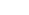}.
Find the number of squares that can be formed with vertices belonging to S and sides parallel to the axes.

## Solution

S = {(x,y) : x,y are non-negative integers}
We calculate number of squares by calculating number of |x| squares ,& number of squares number of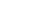squares.
Now number of |x| squares = number of choosing one pair of lines with difference 1 parallel to x axis & integer distance x number of choosing one pair of lines to y axis with distance 1 & integer distance from y axis ==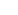Similarly number of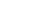squares
=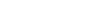So total number of squares
=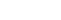=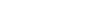This is a Test of Mathematics Solution Subjective 59 (from ISI Entrance). The book, Test of Mathematics at 10+2 Level is Published by East West Press. This problem book is indispensable for the preparation of I.S.I. B.Stat and B.Math Entrance.

Also visit: I.S.I. & C.M.I. Entrance Course of Cheenta

## Problem

Consider the set of point S = { (x,y) : x,y are non-negative integers}.
Find the number of squares that can be formed with vertices belonging to S and sides parallel to the axes.

## Solution

S = {(x,y) : x,y are non-negative integers}
We calculate number of squares by calculating number of |x| squares ,& number of squares number ofsquares.
Now number of |x| squares = number of choosing one pair of lines with difference 1 parallel to x axis & integer distance x number of choosing one pair of lines to y axis with distance 1 & integer distance from y axis ==Similarly number ofsquares
=So total number of squares
==### Knowledge Partner